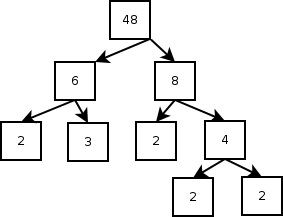# Factorize, More Boxes

Boxes and arrows, I know, it doesn’t look like a program yet. Still, it all is going somewhere, I promise. Let’s take a minute and talk about how factorization works. It’s pretty straightforward: either a number is evenly divisible by some other number (a factor) or it isn’t. Let’s look at an example: 48. What numbers go into 48? Well, I always think of it as 6 times 8, but it’s also 4 times 12. I’m the one with the keyboard, so let’s do 6 times 8. Now, let’s look at 6. Is it evenly divisible by anything? Sure, 2 and 3. How about them? Nope, they are not evenly divisible into smaller integer factors. That’s what it means to be prime, and two and three are the first two prime numbers. Eight, on the other side, divides down into twos. Here’s a tree, where each number gets split down into factors. When a factor can’t be split any more, that factor is prime:The way the program is going to work (refer back to the previous drawing) is to start out with a number to factorize — let’s repeat this example and use 48 — and a list of primes. It’s going to start at the small end of the list of primes and keep trying until it runs out of primes or until it divides the number all the way down to primes. Here’s the tree the program would draw:Here, the program would try the first prime, two. That works, so it would divide 48 by 2 and get 12. It would start over with 12 and see if two goes into 12. It does, and it would try again on 6. That works, too, and it would try again to divide 2 into 3. That doesn’t go evenly, so it would go to the next prime. The next prime is 3, which does go into 3 evenly. 3 divided by 3 is 1, so the program would know that it was done. This kind of drawing is called a tree (it’s upside down, don’t worry about it, but 48 is the root and all the primes are leaves). I’ve colored the primes green to highlight how they’re not being divided, and because they’re the leaves.

This process is pretty darned simple to explain. The only thing missing is, gosh, a list of prime numbers! It’s getting late and once again, I’m going to have to leave that for the next time. Here’s a bit more detail on the flowchart, though. This is the detail for the “let p be the next prime number” box. The first time we run through, we want to start at the circle labeled, “A,” but on all the following times, we want to come in at the circle marked, “B.”